Updating search results...

# 50 Results

View
Selected filters:
• University of Alabama in HuntsvilleUnrestricted Use
CC BY
Rating
2.0 stars

This resource consists of a Java applet and expository text. The applet is a simulation of the ballot experiment: The votes in an election are randomly counted. The event of interest is that the winning candidate is always ahead in the vote count.

Subject:
Statistics and Probability
Material Type:
Activity/Lab
Interactive
Simulation
Provider:
University of Alabama in Huntsville
Provider Set:
Virtual Laboratories in Probability and Statistics
Author:
Kyle Siegrist
02/16/2011Unrestricted Use
CC BY
Rating
0.0 stars

This resource consists of a Java applet and expository text. The applet is a simulation of Bertrand's experiment: a random chord on a circle The event of interest is whether the length of the chord is larger than the length of the inscribed equilateral triangle. Three models for generating the random chord can be used.

Subject:
Statistics and Probability
Material Type:
Activity/Lab
Interactive
Simulation
Provider:
University of Alabama in Huntsville
Provider Set:
Virtual Laboratories in Probability and Statistics
Author:
Kyle Siegrist
02/16/2011Unrestricted Use
CC BY
Rating
0.0 stars

This resource consists of a Java applet and expository text. The applet illustrates Bayesian estimation of the probability of heads for a coin. The prior beta distribution, true probability of heads, and the sample size can be specified. The applet shows the posterior beta distribution.

Subject:
Statistics and Probability
Material Type:
Activity/Lab
Interactive
Simulation
Provider:
University of Alabama in Huntsville
Provider Set:
Virtual Laboratories in Probability and Statistics
Author:
Kyle Siegrist
02/16/2011Unrestricted Use
CC BY
Rating
0.0 stars

This resource consists of a Java applet and expository text. The applet simulates a random sample from a beta distribution, and computes standard point estimates of the left and right parameters. The bias and mean square error are also computed.

Subject:
Statistics and Probability
Material Type:
Activity/Lab
Interactive
Simulation
Provider:
University of Alabama in Huntsville
Provider Set:
Virtual Laboratories in Probability and Statistics
Author:
Kyle Siegrist
02/16/2011Unrestricted Use
CC BY
Rating
0.0 stars

This resource consist of a Java applet and expository text. The applet simulates Bernoulli trials in terms of coin tosses. The random variables of interest are the number of heads and the proportion of heads. The number of coins and the probability of heads can be varied. The applet illustrates the law of large numbers and the central limit theorem.

Subject:
Statistics and Probability
Material Type:
Activity/Lab
Interactive
Simulation
Provider:
University of Alabama in Huntsville
Provider Set:
Virtual Laboratories in Probability and Statistics
Author:
Kyle Siegrist
02/16/2011Unrestricted Use
CC BY
Rating
0.0 stars

This resource consists of a Java applet and expository text. The applet simulates Bernoulli trials in terms of random points on a timeline. The random variables of interest are the number of successes and the proportion of successes. The number of trials and the probability of success can be varied. This applet illustrates the law of large numbers, the central limit theorem, and the binomial distribution.

Subject:
Statistics and Probability
Material Type:
Activity/Lab
Interactive
Simulation
Provider:
University of Alabama in Huntsville
Provider Set:
Virtual Laboratories in Probability and Statistics
Author:
Kyle Siegrist
02/16/2011Unrestricted Use
CC BY
Rating
0.0 stars

This resource consists of a Java applet and expository text. The applet is a simulation of the birthday experiment: a sample of size n is chose at random and with replacement from the first m positive integers. The random variable of interest is the number of distinct sample values. The event of interest is that all sample values are distinct.

Subject:
Statistics and Probability
Material Type:
Activity/Lab
Interactive
Simulation
Provider:
University of Alabama in Huntsville
Provider Set:
Virtual Laboratories in Probability and Statistics
Author:
Kyle Siegrist
02/16/2011Unrestricted Use
CC BY
Rating
0.0 stars

This resource consists of a Java applet and expository text. The applet simulates the bivariate normal distribution. The means are set at 0, but the standard deviations and the correlation can be varied. Simulated points from the distribution are shown as dots in a scatterplot.

Subject:
Statistics and Probability
Material Type:
Activity/Lab
Interactive
Simulation
Provider:
University of Alabama in Huntsville
Provider Set:
Virtual Laboratories in Probability and Statistics
Author:
Kyle Siegrist
02/16/2011Unrestricted Use
CC BY
Rating
0.0 stars

This resource consists of a Java applet and expository text. The Java applet illustrates the bivariate uniform distribution on three types of regions: a square, a circle, and a triangle. Simulated points from the distribution are shown as dots in a scatterplot.

Subject:
Statistics and Probability
Material Type:
Activity/Lab
Interactive
Simulation
Provider:
University of Alabama in Huntsville
Provider Set:
Virtual Laboratories in Probability and Statistics
Author:
Kyle Siegrist
02/16/2011Unrestricted Use
CC BY
Rating
0.0 stars

This resource consists of a Java applet and expository text. The applet simulates Buffon's coin experiment. The radius of the coin can be varied. The applet illustrates a random experiment, the sample space, random variables, events, probability, and relative frequency.

Subject:
Statistics and Probability
Material Type:
Activity/Lab
Interactive
Simulation
Provider:
University of Alabama in Huntsville
Provider Set:
Virtual Laboratories in Probability and Statistics
Author:
Kyle Siegrist
02/16/2011Unrestricted Use
CC BY
Rating
0.0 stars

This resource consists of a Java applet and expository text. The applet simulates Buffon's needle experiment and the corresponding approximation of pi. The event of interest is that the needle crosses a crack. The length of the needle can be varied. The applet illustrates a random experiment, the sample space, random variables, probability, and relative frequency.

Subject:
Statistics and Probability
Material Type:
Activity/Lab
Interactive
Simulation
Provider:
University of Alabama in Huntsville
Provider Set:
Virtual Laboratories in Probability and Statistics
Author:
Kyle Siegrist
02/16/2011Unrestricted Use
CC BY
Rating
0.0 stars

This resource consists of a Java applet and expository text. The applet is a simulation of drawing n cards from a standard deck. The parameter n can be varied.

Subject:
Statistics and Probability
Material Type:
Activity/Lab
Interactive
Simulation
Provider:
University of Alabama in Huntsville
Provider Set:
Virtual Laboratories in Probability and Statistics
Author:
Kyle Siegrist
02/16/2011Unrestricted Use
CC BY
Rating
0.0 stars

This resource consists of a Java applet and expository text. The applet simulates the chuck-a-luck experiment of rolling 3 fair dice. The random variable of interest is the net profit of the player.

Subject:
Statistics and Probability
Material Type:
Activity/Lab
Interactive
Simulation
Provider:
University of Alabama in Huntsville
Provider Set:
Virtual Laboratories in Probability and Statistics
Author:
Kyle Siegrist
02/16/2011Unrestricted Use
CC BY
Rating
0.0 stars

This resource consists of a Java applet and expository text. The applet is a simulation of the experiment that consists of tossing a coin and then rolling either a red die or a green die, depending on the outcome of the coin toss. The probability of heads and the distributions of the two dice can be specified. The applet illustrates a two-stage experiment.

Subject:
Statistics and Probability
Material Type:
Activity/Lab
Interactive
Simulation
Provider:
University of Alabama in Huntsville
Provider Set:
Virtual Laboratories in Probability and Statistics
Author:
Kyle Siegrist
02/16/2011Unrestricted Use
CC BY
Rating
0.0 stars

This resource consists of a Java applet and expository text. The applet simulates coin tosses. The number of coins and the probability of heads can be varied.

Subject:
Statistics and Probability
Material Type:
Activity/Lab
Simulation
Provider:
University of Alabama in Huntsville
Provider Set:
Virtual Laboratories in Probability and Statistics
Author:
Kyle Siegrist
01/18/2006Unrestricted Use
CC BY
Rating
0.0 stars

This resource consists of a Java applet and expository text. The applet is a simulation of the coupon collector problem, which consists of sampling from the first m positive integers, with replacement, until k distinct values are obtained. The parameters m and k can be varied. The random variable of interest is the size of the sample.

Subject:
Statistics and Probability
Material Type:
Activity/Lab
Interactive
Simulation
Provider:
University of Alabama in Huntsville
Provider Set:
Virtual Laboratories in Probability and Statistics
Author:
Kyle Siegrist
02/16/2011Unrestricted Use
CC BY
Rating
0.0 stars

This resource consists of a Java applet and expository text. The applet is a simulation of the standard casino craps game. The random variables of interest are the winning under various bets.

Subject:
Statistics and Probability
Material Type:
Activity/Lab
Interactive
Simulation
Provider:
University of Alabama in Huntsville
Provider Set:
Virtual Laboratories in Probability and Statistics
Author:
Kyle Siegrist
02/16/2011Unrestricted Use
CC BY
Rating
0.0 stars

This resource consists of a Java applet and expository text. The applet simulates rolling n dice. The random variables of interest are the sum of the scores, the maximum score, the minimum score, and the number of aces. The number of dice and the probability distribution that governs the dice can be specified. The applet illustrates various distributions and the central limit theorem.

Subject:
Statistics and Probability
Material Type:
Activity/Lab
Interactive
Simulation
Provider:
University of Alabama in Huntsville
Provider Set:
Virtual Laboratories in Probability and Statistics
Author:
Kyle Siegrist
02/16/2011Unrestricted Use
CC BY
Rating
0.0 stars

This resource consists of a Java applet and expository text. The applet simulates the rolling of n dice. The number of dice and the probability distribution that governs the dice can be specified.

Subject:
Statistics and Probability
Material Type:
Activity/Lab
Interactive
Simulation
Provider:
University of Alabama in Huntsville
Provider Set:
Virtual Laboratories in Probability and Statistics
Author:
Kyle Siegrist
02/16/2011Unrestricted Use
CC BY
Rating
0.0 stars

This resource consists of a Java applet and expository text. The applet simulates the experiment of rolling a die and then tossing a coin the number of times shown on the die. The die distribution and the probability of heads can be specified. The applet illustrates a two-stage experiment.

Subject:
Statistics and Probability
Material Type:
Activity/Lab
Interactive
Simulation
Provider:
University of Alabama in Huntsville
Provider Set:
Virtual Laboratories in Probability and Statistics
Author:
Kyle Siegrist
02/16/2011Unrestricted Use
CC BY
Rating
0.0 stars

This resource consists of a Java applet and expository text. The applet is a simulation of the experiment of selecting n objects at random from the first m positive integers. The random variables of interest are the order statistics. The applet illustrates the distributions of the order statistics.

Subject:
Statistics and Probability
Material Type:
Activity/Lab
Interactive
Simulation
Provider:
University of Alabama in Huntsville
Provider Set:
Virtual Laboratories in Probability and Statistics
Author:
Kyle Siegrist
02/16/2011Unrestricted Use
CC BY
Rating
0.0 stars

This resource consists of a Java applet and expository text. The applet is a simulation of the fire process, an interacting particle system consisting of a rectangular lattice of "trees". At each discrete time unit, each tree is either on green, on fire, or burnt. A tree on fire at a given time can catch neighboring trees on fire at the next time unit. The probabilities of fire spread can be varied.

Subject:
Statistics and Probability
Material Type:
Activity/Lab
Interactive
Simulation
Provider:
University of Alabama in Huntsville
Provider Set:
Virtual Laboratories in Probability and Statistics
Author:
Kyle Siegrist
02/16/2011Unrestricted Use
CC BY
Rating
0.0 stars

This resource consists of a Java applet and descriptive text. The applet illustrates Bernoulli trials in terms of a Galton Board. The number of trials and the probability of success can be varied. The random variables of interest are the number of successes and the probability of a success. The applet illustrates the binomial distribution, the law of large numbers and the central limit theorem.

Subject:
Statistics and Probability
Material Type:
Activity/Lab
Interactive
Simulation
Provider:
University of Alabama in Huntsville
Provider Set:
Virtual Laboratories in Probability and Statistics
Author:
Kyle Siegrist
02/16/2011Unrestricted Use
CC BY
Rating
0.0 stars

This resource consists of a Java applet and expository text. The applet illustrates the Galton board in which a ball falls through a triangular array of pegs. Various combinatorial ideas are illustrated, including combinations, bit strings, and binomial coefficients.

Subject:
Statistics and Probability
Material Type:
Activity/Lab
Interactive
Simulation
Provider:
University of Alabama in Huntsville
Provider Set:
Virtual Laboratories in Probability and Statistics
Author:
Kyle Siegrist
02/16/2011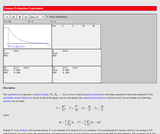Unrestricted Use
CC BY
Rating
0.0 stars

This resource consists of a Java applet and expository text. The applet simulates a random sample from a gamma distribution, and computes standard point estimates of the shape and scale parameters. The bias and mean square error are also computed.

Subject:
Statistics and Probability
Material Type:
Activity/Lab
Interactive
Simulation
Provider:
University of Alabama in Huntsville
Provider Set:
Virtual Laboratories in Probability and Statistics
Author:
Kyle Siegrist
02/16/2011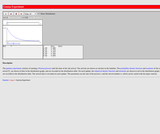Unrestricted Use
CC BY
Rating
0.0 stars

This resource consists of a Java applet and expository text. The applet simulates the time of the k'th arrival in a Poisson process. The arrival number k and the rate of the Poisson process can be varied. The applet illustrates the gamma distribution and a special case of the central limit theorem.

Subject:
Statistics and Probability
Material Type:
Activity/Lab
Simulation
Provider:
University of Alabama in Huntsville
Provider Set:
Virtual Laboratories in Probability and Statistics
Author:
Kyle Siegrist
02/01/2006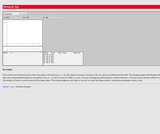Unrestricted Use
CC BY
Rating
0.0 stars

This resource consists of a Java applet and expository text. The applet allows the user to construct a histogram by clicking on a number line to generate the sample data. The class width can be varied and various summary statistics can be displayed.

Subject:
Statistics and Probability
Material Type:
Activity/Lab
Simulation
Provider:
University of Alabama in Huntsville
Provider Set:
Virtual Laboratories in Probability and Statistics
Author:
Kyle Siegrist
02/20/2006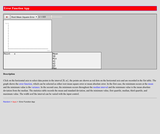Unrestricted Use
CC BY
Rating
0.0 stars

This resource consists of a Java applet and expository text. The applet allows the user to construct a histogram by clicking on a number line to generate the sample data. The graph of an error function is shown, either mean square error or mean absolute error.

Subject:
Statistics and Probability
Material Type:
Interactive
Provider:
University of Alabama in Huntsville
Provider Set:
Virtual Laboratories in Probability and Statistics
Author:
Kyle Siegrist
11/04/2014Unrestricted Use
CC BY
Rating
0.0 stars

This resource consists of a Java applet and expository text. The applet allows the user to construct a scatterplot by clicking on a graph to generate the bivariate data. The means, standard deviations, correlation, and the regression line are shown.

Subject:
Statistics and Probability
Material Type:
Activity/Lab
Interactive
Simulation
Provider:
University of Alabama in Huntsville
Provider Set:
Virtual Laboratories in Probability and Statistics
Author:
Kyle Siegrist
02/16/2011Unrestricted Use
CC BY
Rating
0.0 stars

This resource consists of a Java applet and expository text. The applet is a simulation of the matching experiment, which consists of a random permutation of the first n positive integers. The random variable of interest is the number of matches (the number of integers in the random permutation that are in their correct positions). The applet illustrates the distribution of the number of matches and the convergence to the Poisson distribution.

Subject:
Statistics and Probability
Material Type:
Activity/Lab
Interactive
Simulation
Provider:
University of Alabama in Huntsville
Provider Set:
Virtual Laboratories in Probability and Statistics
Author:
Kyle Siegrist
02/16/2011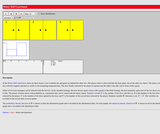Unrestricted Use
CC BY
Rating
0.0 stars

This resource consists of a Java applet and expository text. The applet is a simulation of the Monty Hall experiment: a car is behind one door, goats are behind the other two doors. The player chooses a door and then the host opens another door. The player is given the option of switching to the remaining door. The stochastic behavior of the host and the probability of the player switching can be specified.

Subject:
Statistics and Probability
Material Type:
Activity/Lab
Simulation
Provider:
University of Alabama in Huntsville
Provider Set:
Virtual Laboratories in Probability and Statistics
Author:
Kyle Siegrist
01/18/2006Unrestricted Use
CC BY
Rating
0.0 stars

This resource consists of a Java applet and expository text. The applet consists of the Monty Hall game: a car is behind one door and goats are behind the other two doors. The player makes an initial choice and the host opens a different door. The player is then given the option of switching to the remaining door. The stochastic behavior of the host can be specified.

Subject:
Statistics and Probability
Material Type:
Activity/Lab
Interactive
Simulation
Provider:
University of Alabama in Huntsville
Provider Set:
Virtual Laboratories in Probability and Statistics
Author:
Kyle Siegrist
02/16/2011Unrestricted Use
CC BY
Rating
0.0 stars

This resource consists of a Java applet and descriptive text. The applet illustrates the number of trials needed to get a specified number of successes in Bernoulli trials, in terms of random points on a discrete timeline. The applet illustrates the negative binomial distribution. The number of successes and the probability of success can be varied.

Subject:
Statistics and Probability
Material Type:
Activity/Lab
Interactive
Simulation
Provider:
University of Alabama in Huntsville
Provider Set:
Virtual Laboratories in Probability and Statistics
Author:
Kyle Siegrist
02/16/2011Unrestricted Use
CC BY
Rating
0.0 stars

This resource consists of a Java applet and expository text. The applet simulates a random sample from a normal distribution, and computes standard point estimates of the distribution mean and standard deviation. The bias and mean square error are also computed.

Subject:
Statistics and Probability
Material Type:
Activity/Lab
Interactive
Simulation
Provider:
University of Alabama in Huntsville
Provider Set:
Virtual Laboratories in Probability and Statistics
Author:
Kyle Siegrist
02/16/2011Unrestricted Use
CC BY
Rating
0.0 stars

This resource consists of a Java applet and expository text. The applet simulates the order statistics of a random sample from a given distribution. The sample size, order, and sampling distribution can be specified.

Subject:
Statistics and Probability
Material Type:
Activity/Lab
Interactive
Simulation
Provider:
University of Alabama in Huntsville
Provider Set:
Virtual Laboratories in Probability and Statistics
Author:
Kyle Siegrist
02/16/2011Unrestricted Use
CC BY
Rating
0.0 stars

This resource consists of a Java applet and expository text. The applet simulates a random sample from a Pareto distribution, and computes standard point estimates of the shape parameter. The bias and mean square error are also computed.

Subject:
Statistics and Probability
Material Type:
Activity/Lab
Interactive
Simulation
Provider:
University of Alabama in Huntsville
Provider Set:
Virtual Laboratories in Probability and Statistics
Author:
Kyle Siegrist
02/16/2011Unrestricted Use
CC BY
Rating
0.0 stars

This resource consists of a Java applet and expository text. The applet simulates the arrivals in a Poisson process during a time interval. The length of the interval and the rate of the process can be varied. The applet illustrates the Poisson distribution and a special case of the central limit theorem.

Subject:
Statistics and Probability
Material Type:
Activity/Lab
Interactive
Simulation
Provider:
University of Alabama in Huntsville
Provider Set:
Virtual Laboratories in Probability and Statistics
Author:
Kyle Siegrist
02/16/2011Unrestricted Use
CC BY
Rating
0.0 stars

This resource consists of a Java applet and expository text. The experiment consists of rolling 5 fair dice. The random variable of interest is the type of hand.

Subject:
Statistics and Probability
Material Type:
Activity/Lab
Interactive
Simulation
Provider:
University of Alabama in Huntsville
Provider Set:
Virtual Laboratories in Probability and Statistics
Author:
Kyle Siegrist
02/16/2011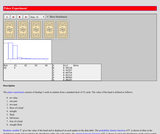Unrestricted Use
CC BY
Rating
0.0 stars

This resource consists of a Java applet and expository text. The applet simulates the basic poker experiment of dealing 5 cards from a standard deck. The random variable of interest is the type of hand.

Subject:
Statistics and Probability
Material Type:
Interactive
Provider:
University of Alabama in Huntsville
Provider Set:
Virtual Laboratories in Probability and Statistics
Author:
Kyle Siegrist
11/04/2014Unrestricted Use
CC BY
Rating
0.0 stars

This resource consists of a Java applet and Expository text. The applet simulates the probability plot that compares the empirical quantiles of a sample from a sampling distribution to the distribution quantiles of a test distribution. The sampling distribution, test distribution, and sample size can be specified.

Subject:
Statistics and Probability
Material Type:
Activity/Lab
Interactive
Simulation
Provider:
University of Alabama in Huntsville
Provider Set:
Virtual Laboratories in Probability and Statistics
Author:
Kyle Siegrist
02/16/2011Unrestricted Use
CC BY
Rating
0.0 stars

This resource consists of a Java applet and descriptive text. The applet illustrates the problem of points: a coin is tossed until either n heads occurs or m tails occurs. The parameters n, m, and the probability of heads can be varied.

Subject:
Statistics and Probability
Material Type:
Activity/Lab
Interactive
Simulation
Provider:
University of Alabama in Huntsville
Provider Set:
Virtual Laboratories in Probability and Statistics
Author:
Kyle Siegrist
02/16/2011Unrestricted Use
CC BY
Rating
0.0 stars

This resource consists of a Java applet and descriptive text. The applet illustrates a random walk on a discrete time interval from 0 to n. The time n and the probability of a step to the right can be varied. The random variables of interest are the final position, the maximum position, and the time of the last zero.

Subject:
Statistics and Probability
Material Type:
Activity/Lab
Interactive
Simulation
Provider:
University of Alabama in Huntsville
Provider Set:
Virtual Laboratories in Probability and Statistics
Author:
Kyle Siegrist
02/16/2011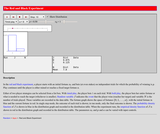Unrestricted Use
CC BY
Rating
0.0 stars

This resource consists of a Java applet and expository text. The applet simulates the red and black experiment in which a player bets on a sequence of Bernoulli trials until a target fortune is reached or the player is ruined. The initial fortune, target fortune, and trial win probability can be varied, and the user can select either of two basic strategies: timid play or bold play.

Subject:
Statistics and Probability
Material Type:
Interactive
Provider:
University of Alabama in Huntsville
Provider Set:
Virtual Laboratories in Probability and Statistics
Author:
Kyle Siegrist
11/04/2014Unrestricted Use
CC BY
Rating
0.0 stars

This resource consists of a Java applet and expository text. The applet allows the student to play the game of red and black, by betting on a sequence of Bernoulli trials until either a target is reached or the player is ruined. The target, initial fortune, and trial win probability can be varied.

Subject:
Statistics and Probability
Material Type:
Activity/Lab
Interactive
Simulation
Provider:
University of Alabama in Huntsville
Provider Set:
Virtual Laboratories in Probability and Statistics
Author:
Kyle Siegrist
02/16/2011Unrestricted Use
CC BY
Rating
0.0 stars

This resource consists of a Java applet and expository text. The applet is a simulation of the standard casino roulette game. The random variables of interest are the winning under various bets.

Subject:
Statistics and Probability
Material Type:
Activity/Lab
Interactive
Simulation
Provider:
University of Alabama in Huntsville
Provider Set:
Virtual Laboratories in Probability and Statistics
Author:
Kyle Siegrist
02/16/2011Unrestricted Use
CC BY
Rating
0.0 stars

The resource consists of a Java applet and expository text. The applet illustrates the distribution of the sample mean of a random sample from a given distribution. The sample size and the sampling distribution can be specified. The applet illustrates the central limit theorem.

Subject:
Statistics and Probability
Material Type:
Activity/Lab
Interactive
Simulation
Provider:
University of Alabama in Huntsville
Provider Set:
Virtual Laboratories in Probability and Statistics
Author:
Kyle Siegrist
02/16/2011Unrestricted Use
CC BY
Rating
0.0 stars

This resource consists of a Java applet and expository text. The applet illustrates the triangle experiment: A stick is broken randomly into three parts. The events of interest are that the pieces form an acute triangle, an obtuse triangle, or no triangle.

Subject:
Statistics and Probability
Material Type:
Activity/Lab
Interactive
Simulation
Provider:
University of Alabama in Huntsville
Provider Set:
Virtual Laboratories in Probability and Statistics
Author:
Kyle Siegrist
02/16/2011Unrestricted Use
CC BY
Rating
0.0 stars

This resource consists of a Java applet and expository text. The applet illustrates the arrivals in a Poisson process where each arrival, independently, is one of two types. The time interval, the rate of the process, and the probability that an arrival is type 1 can be varied. The applet illustrates "splitting" a Poisson process.

Subject:
Statistics and Probability
Material Type:
Activity/Lab
Interactive
Simulation
Provider:
University of Alabama in Huntsville
Provider Set:
Virtual Laboratories in Probability and Statistics
Author:
Kyle Siegrist
02/16/2011Unrestricted Use
CC BY
Rating
0.0 stars

This resource consists of a Java applet and expository text. The applet simulates a random sample from the uniform distribution on the interval [0,a], and computes standard point estimates of a. The bias and mean square error are also computed.

Subject:
Statistics and Probability
Material Type:
Activity/Lab
Interactive
Simulation
Provider:
University of Alabama in Huntsville
Provider Set:
Virtual Laboratories in Probability and Statistics
Author:
Kyle Siegrist
02/16/2011Unrestricted Use
CC BY
Rating
0.0 stars

This resource consists of a Java applet and expository text. The applet is a simulation of the voter experiment, an interacting partile system that consists of a rectangular array of sites. At each discrete time unit, a site is chosen at random, a neighboring site is chosen, and the color of the first site is changed to that of the neighbor. The applet illustrates clustering of the colors and convergence to consensus.

Subject:
Statistics and Probability
Material Type:
Activity/Lab
Interactive
Simulation
Provider:
University of Alabama in Huntsville
Provider Set:
Virtual Laboratories in Probability and Statistics
Author:
Kyle Siegrist